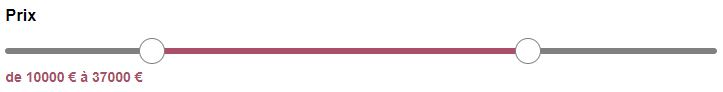Maxy.fr

When designing HTML forms, you may quickly find yourself limited by the basic form elements provided. For example, if you use sliders with the `<input type="range">` element, they only allow the use of a single slider per element. However, there may be times when you need a field with two sliders to select a minimum and maximum value, such as for choosing a price range in a search form.

## Double Slider Field

This is a problem I faced and for which I developed a solution to easily create a double slider field like this:Implementation is quite simple. You just need to include the corresponding JavaScript and CSS files in your project (available in the GitHub repository) and use the following HTML code:

```<!DOCTYPE html>
<html>
<title>Double Range Example</title>
<body>

<form>
<div id="doubleRange" class="doubleRange">
<div class="barre">
<div class="barreMilieu" style="width:50%; left:25%;"></div>
<div class="t1 thumb" style="left:25%"></div>
<div class="t2 thumb" style="left:75%;"></div>
</div>
<div class="label">de <span class="labelMin"></span> à <span class="labelMax"></span></div>
<input type="hidden" name="pmin" value="" class="inputMin"/>
<input type="hidden" name="pmax" value="" class="inputMax"/>
</div>
</form>

<script type="text/javascript" src="doubleRange.js"></script>
<script type="text/javascript">
setDoubleRange({
element: '#doubleRange',
minValue: 0,
maxValue: 50000,
maxInfinite: true,
stepValue: 1000,
defaultMinValue: 500,
defaultMaxValue: 10000,
unite: '€'
});
</script>
</body>
</html>```

The `<div>` element with the ID `#doubleRange` contains the double slider. Labels allow real-time display of the selected values. The values are returned to the form via the `pmin` and `pmax` fields in this example.

## Configuration

The JavaScript code allows you to set up the double slider. It can be configured using the following options:

• element: This is the ID (#element) or class (.element) of the HTML element containing the double slider (in the example, #doubleRange). If you want to use multiple double sliders, you can duplicate the code using unique IDs or classes.
• minValue: This is the minimum value that can be selected.
• maxValue: This is the maximum value that can be selected.
• maxInfinite: Possible values are true or false. If you set it to true, the maximum position of the slider will be replaced with infinity, and the value of the `pmax` field will be empty. Set it to false to disable this feature.
• stepValue: This is the step value for the slider. For example, if you set it to 5, the slider will move in increments of 5.
• defaultMinValue: Here, you can specify the default value for the first slider when the page loads.
• defaultMaxValue: Here, you can specify the default value for the second slider when the page loads.
• unite: This is the unit to be displayed in the labels for the real-time display of values.

You now have all the elements you need to use the double slider. The tool is available as open source on GitHub and may be further improved in the future if needed.

# View the GitHub repository for double range

Categories : Javascript, HTML

By Guillaume - 11/13/2020 at 03:44 pm